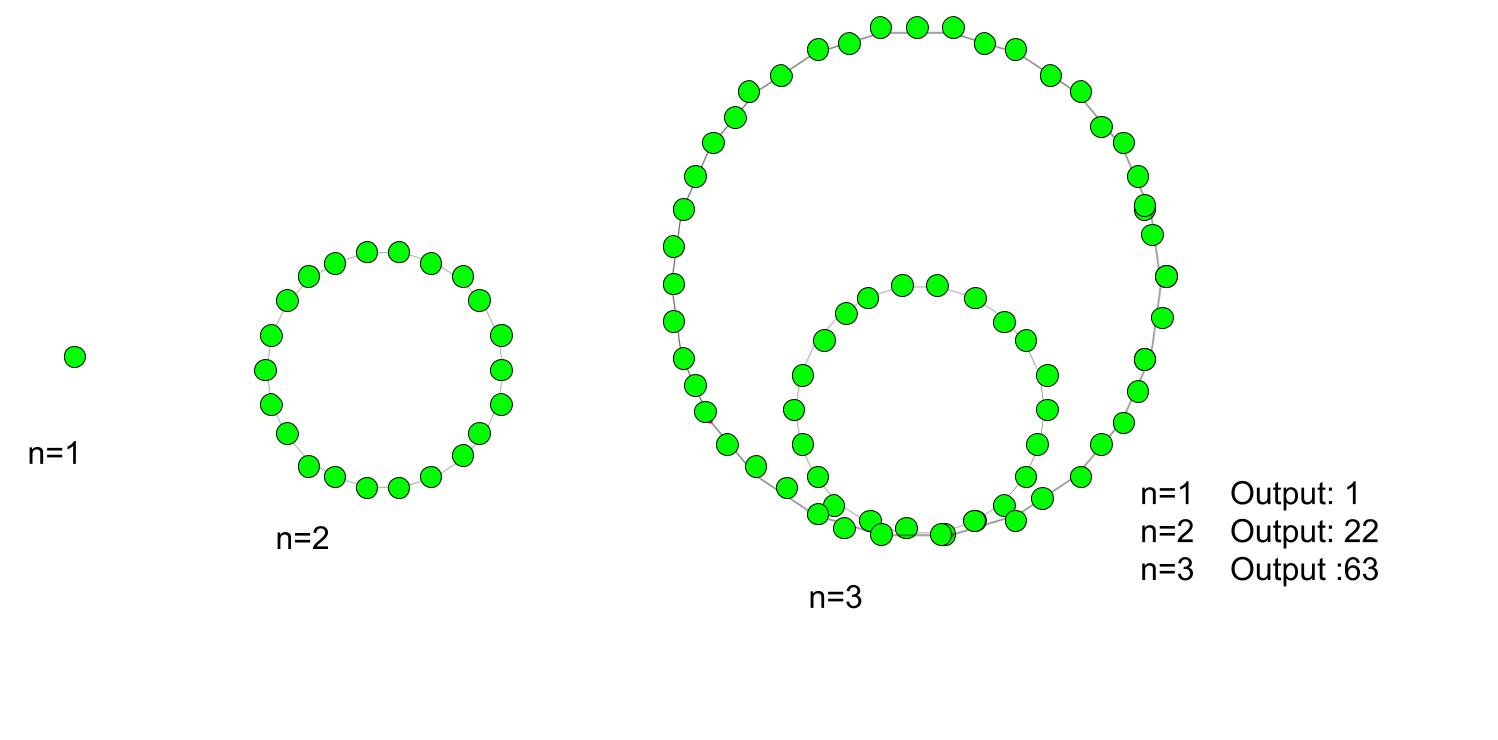# Icosidigonal number

Given a number n, the task is to find the nth Icosidigonal number (Isdn).
The polygon has the many gons, depends on their gonal number series. In mathematics, there is a number of gonal numbers and the icosidigonal number is one of them and these numbers have 22 -sided polygon (icosidigon). An icosidigonal number belong to the class of figurative number. They have one common dots points and other dots pattern is arranged in an n-th nested icosidigon pattern.

Examples :

Input : 2
Output :22

Input :6
Output :306Formula for nth Icosidigonal number:## C++

 // C++ program to find nth Icosidigonal  // number  #include  using namespace std;     // Function to calculate Icosidigonal number  int icosidigonal_num(long int n)  {      // Formula for finding      // nth Icosidigonal number      return (20 * n * n - 18 * n) / 2;  }     // Driver function  int main()  {      long int n = 4;      cout << n << "th Icosidigonal number :" << icosidigonal_num(n);      cout << endl;      n = 8;      cout << n << "th Icosidigonal number:" << icosidigonal_num(n);      return 0;  }

## Java

 // Java program to find nth   // Icosidigonal number   import java.io.*;     class GFG   {             // Function to calculate       // Icosidigonal number      static int icosidigonal_num(int n)       {                 // Formula for finding      // nth Icosidigonal number             return (20 * n * n - 18 * n) / 2;      }     // Driver Code  public static void main (String[] args)   {         int n = 4;      System.out.print (n + "th Icosidigonal number :");      System.out.println (icosidigonal_num(n));         n = 8;      System.out.print (n + "th Icosidigonal number :");      System.out.println (icosidigonal_num(n));  }  }     // This code is contributed by ajit

## Python 3

 # python 3 program to find   # nth Icosidigonal number     # Function to calculate  # Icosidigonal number  def icosidigonal_num(n) :             # Formula for finding      # nth Icosidigonal number      return (20 * n * n -              18 * n) // 2    # Driver Code  if __name__ == '__main__' :                 n = 4     print(n,"th Icosidigonal " +                     "number : ",               icosidigonal_num(n))      n = 8     print(n,"th Icosidigonal " +                     "number : ",               icosidigonal_num(n))         # This code is contributed m_kit

## C#

 // C# program to find nth   // Icosidigonal number   using System;     class GFG  {             // Function to calculate       // Icosidigonal number      static int icosidigonal_num(int n)       {                 // Formula for finding      // nth Icosidigonal number      return (20 * n * n -               18 * n) / 2;      }     // Driver Code  static public void Main ()  {  int n = 4;  Console.Write(n + "th Icosidigonal " +                            "number :");  Console.WriteLine(icosidigonal_num(n));     n = 8;  Console.Write (n + "th Icosidigonal "+                            "number :");  Console.WriteLine(icosidigonal_num(n));  }  }     // This code is contributed by ajit

## PHP

 

Output :

4th Icosidigonal number :124
8th Icosidigonal number:568


Don’t stop now and take your learning to the next level. Learn all the important concepts of Data Structures and Algorithms with the help of the most trusted course: DSA Self Paced. Become industry ready at a student-friendly price.

My Personal Notes arrow_drop_upCheck out this Author's contributed articles.

If you like GeeksforGeeks and would like to contribute, you can also write an article using contribute.geeksforgeeks.org or mail your article to contribute@geeksforgeeks.org. See your article appearing on the GeeksforGeeks main page and help other Geeks.

Please Improve this article if you find anything incorrect by clicking on the "Improve Article" button below.

Improved By : jit_t